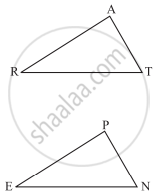# You Want to Show that δArt ≅ δPen, If It is Given that ∠T = ∠N and You Are to Use Sas Criterion, You Need to Have - Mathematics

Sum

You want to show that ΔART ≅ ΔPEN,

If it is given that ∠T = ∠N and you are to use SAS criterion, you need to have

1) RT = and

2) PN =#### Solution

1) RT = EN

2) PN = AT

Concept: Criteria for Congruence of Triangles
Is there an error in this question or solution?

#### APPEARS IN

NCERT Class 7 Maths
Chapter 7 Congruence of Triangles
Exercise 7.2 | Q 2.2 | Page 149